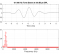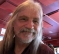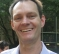## Is RMS a good metric to estimate gainStarted by 3 years ago8 replieslatest reply 3 years ago310 views

I have two vectors V1 and V2. V1 is the source signal vector and V2 being the (possibly) degraded (attenuated/amplified) output signal. I have calculated the RMS of both V1 and V2. Now, I was wondering if the ratio RMS(V2)/RMS(V1) is a good estimation or approximation of the gain. Can I use this metric as a gain factor?

[ - ]You could use this an indicator gain in dB:  20*log10( RMS(V2) / RMS(V1) ).

[ - ]This looks really good (and more inclined towards textbook definition of gain). Is this independent of factors like DC components and noise? I'm looking to apply this for recorded audio.

[ - ]Yes, why not?

BR

Mariano

[ - ]RMS reflects power but for gain it is either used as ratio of output/input or adjusted for bitwidth such as dBFs which is 10log(power/fullscale power) and so removes effect of bitwidth.

[ - ]By "vector", I presume you mean a finite sequence $\{ v[n] \}$ of $N$ samples of a signal and RMS is

$$V_\mathrm{rms} \triangleq \sqrt{\frac{1}{N} \sum\limits_{n=0}^{N-1} |v[n]|^2}$$

To use that ratio as a measure of gain, you may want to consider how "representative" your source signal is.  Say, if it was simply a sinusoid (having some frequency), the ratio of RMS of output to RMS of source would be a good measure of gain for that specific frequency.  The gain might be different for a sinusoid of a different frequency.

You might want the source signal to be more broadbanded than a single sinusoid.

And also consider that there may be a constant DC offset in the output signal that would add to the RMS of the output.  So you might want to measure the output when the input is zero to learn how much any constant DC offset might be.  You might want to subtract that DC offset squared from the squares of the output samples before mean-rooting.

[ - ]This is an interesting and informative perspective. That's correct. I am looking at audio samples, which is more often than not a speech signal and hence a combination of different sinusoids of different frequencies, and when the output is typically a similar case probably with (possibly) missing frequencies or noise added.

So, if I got it right, according to the your answer, (RMS(V2) - RMS(before-V2))/RMS(V1) is the best way, correct?

[ - ]